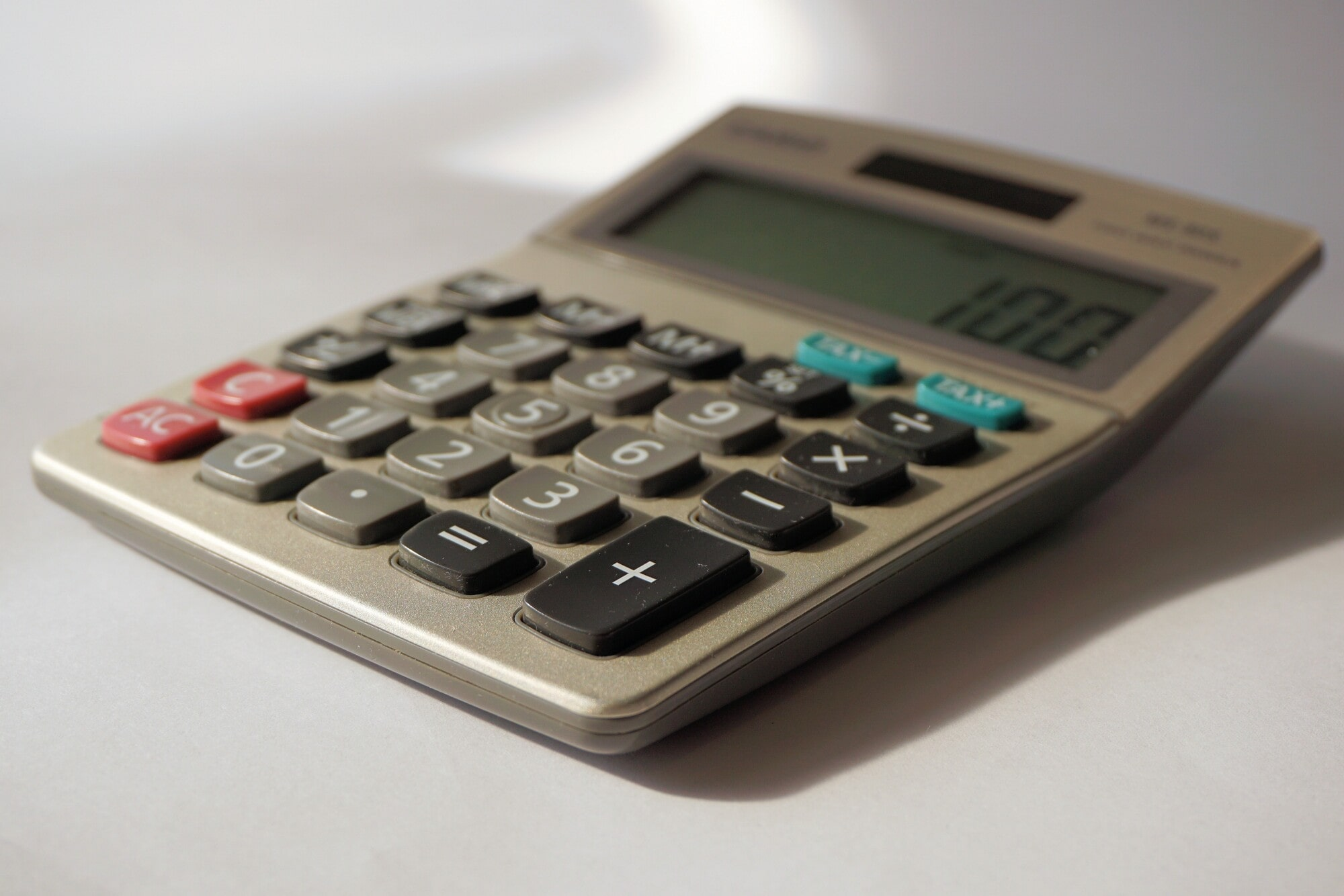Skip to main contentDid you know that the median gross rent in San Diego is \$1,885?

If you're a landlord in San Diego County, it's important to know how to calculate prorated rent. Prorated rent refers to the amount of rent a tenant pays for a partial rental period, such as when they move in or move out in the middle of the month.

Understanding how to calculate this can help you ensure that your tenants are paying a fair amount of rent and that you're not losing out on rent payments. Keep reading to learn how to calculate prorated rent.

## Making Calculations

The first step in calculating prorated rent is to determine the tenant's daily rental rate. First, you have to divide the monthly rent by the month's total number of days. For example, if the monthly rent is \$1,500 and there are 30 days in the month, the daily rental rate would be \$50 (\$1,500 divided by 30 = \$50).

After that, you need to figure out the number of days that the tenant will occupy the rental unit during the partial rental period. For instance, if the tenant is moving in on the 15th and the rental period starts on the 1st of the month, the tenant will occupy the rental unit for 16 days. This is due to counting from the 15th to the end of the month.

To calculate the prorated rent, you multiply the daily rental rate by the number of days the tenant will occupy the rental unit during the partial rental period. In the example above, the prorated rent for the tenant moving in on the 15th of the month would be \$800 (\$50 times 16 = \$800).

## When Should I Calculate Prorated Tenant Rates?

It's important to note that you can calculate prorated rent for both move-in and move-out situations. When a tenant moves out in the middle of the month, you would use the same formula to determine the number of days they occupied the rental unit and then subtract that from the total number of days in the month. This will give you the number of days the unit was unoccupied.

You would then finish the rent calculation based on the number of days the tenant occupied the unit.

## Keep the Rental Market in Mind

It's also worth mentioning that the rental market in San Diego County can impact prorated rent. If there's high demand for rental properties and low availability, your landlord could charge higher rates and may not need the option of prorated rent.

In the case of a slow rental market, landlords should offer prorated rent to entice tenants into renting their properties.

To make the process of calculating prorated rent easier, some landlords use rent calculation software or rent payment portals that automate the process.

## Now You Know How to Calculate Prorated Rent

Now that you've learned how to calculate prorated rent, you can feel more confident and avoid getting less than you deserve.

McLain Properties can offer you comprehensive property management solutions. From rent calculation to tenant screening, feel free to ask about our services.

back